• Search Icon

# How to do your own crop estimate

March 2022

 KOBIE DE BEER, FORMER MANAGER, INSURANCE SERVICES (HARVEST), SANTAM AGRICULTUREUsing the following information as a guideline, you can estimate your potential crop yield and possible income for the season. Keep in mind that there are many variables that can affect the accuracy of a crop or yield estimate. The closer you come to harvest time, the more accurate are the determinations that can be made, because the chance is smaller of major variables occurring.

An area that is studied in depth is the determination of crop yields or crop estimates. Certain formulae are developed and adjusted, but a thorough knowledge of, among other things, the farming branch and region remains important in determining a crop yield.

One of the most critical factors is the kernel mass. It not only varies considerably from one season to the next, but also within one season. Even after the crop is mature, factors like ear rot and other pathogens can affect the kernel mass. The guidelines are as follows:

WHEAT
With wheat a good average seed count is approximately:

• Dryland – 3 500 seeds/100 g
• Irrigation – 3 000 seeds/100 g

Two general practices, sowing and planting, are described separately to determine yields.

Sowing or planting wheat in very narrow rows

1. Firstly, the number of ears or stalks per square metre (m²) are determined.
2. The average number of seeds per ear are determined by counting the seeds in a sample of wheat ears, including small and big ears in proportion. If the seeds cannot yet be counted, a good average count for the cultivar concerned can be used.
3. The following formula can be used to calculate the yield where wheat was sown: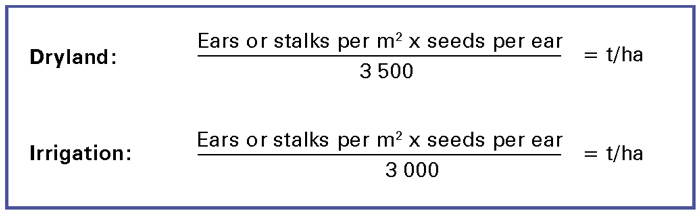Wheat planted in distinguishable rows

1. Determine the row width as follows: The distance over 10 row spaces is measured, in other words from row 1 to row 11, and the distance thus obtained is divided by 10 to determine the row width.2. The number of ears or stalks in the row over a distance of 3 m is counted.
3. The number of seeds per ear is determined by counting a sample of ears, which includes small and large ears in proportion. If the seeds cannot yet be counted, a good average count for the cultivar concerned can be used.
4. The formula below can be used to calculate the yield where wheat has been planted in distinguishable rows.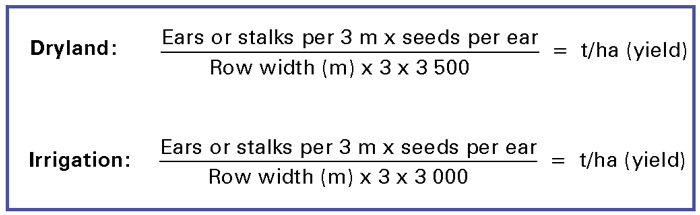MAIZE

1. A guideline of 0,28 g/kernel can be used for the average kernel mass of maize where the seeds can be counted in order to calculate the potential crop.
2. When the seeds cannot be counted, 120 g to 180 g per ear, depending on the population and occurrence of multiple ears, can be used.
3. First determine the average number of ears per 10 m. When the plants are in the vegetative stage, factors like the population, multiple ears and the sprouting ability of the plants must be taken into account.
4. Determine the average mass in gram per ear.
5. The formula below can now be used to calculate the yield.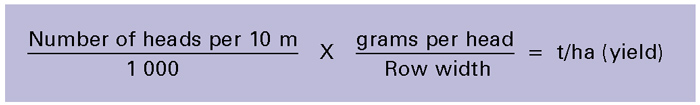SUNFLOWER

1. Use the following guidelines to determine the average seed mass per head at a young stage before the seeds on the head can be counted.
2.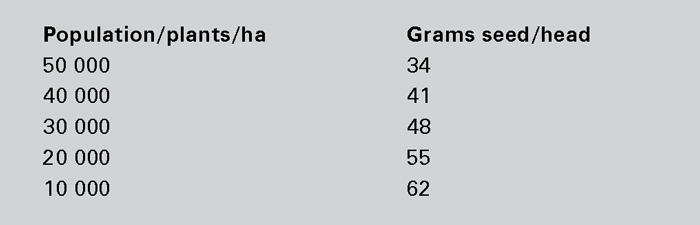Use the following method to count the seeds per head:
• Measure the diameter of the head.
• Measure the diameter of the centre seed set if applicable.
• Count the number of seeds per cm2 and divide this number by 10 to obtain the number of seeds per cm2
• Calculate the productive area as follows: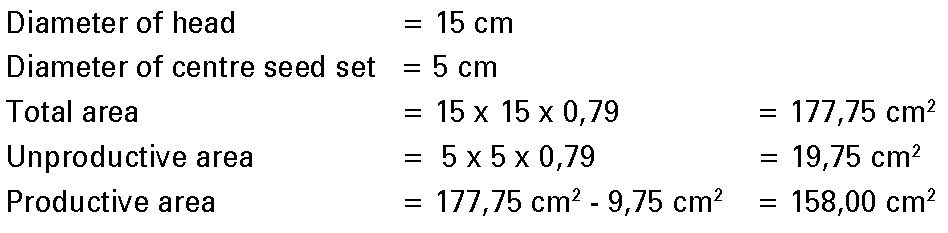• Multiply the number of seeds per square centimetre (cm2) by the productive area to get the number of seeds per head.
• Multiply the number of seeds on the head with the average mass per seed to obtain the mass of the head. Use a mass of 0,04 g per seed as guideline.
3. Count the number of heads per 10 m.
4. Measure the row width.
5. Use the following formula to calculate the yield:DRY BEANS AND SOYBEANS
Follow the steps below to do a crop estimate for dry beans and soybeans:

1. Determine the number of plants per 10 m and the average row width.2. Determine the average number of pods per plant and seeds per pod.
Soybeans, ± 1,8 seeds/pod: Mass: ± 0,16 g/seed
Dry beans: Seeds/pod and mass/seed vary according to cultivar:
Small white canning types: Mass ± 0,19 g/seed
Red speckled types: Mass ± 0,47 g/seed
3. Allocate a mass per plant using the above guidelines, but always keep the following in mind:
• Evenness of plant population and plant height.
• General appearance and colour of the plants.
• Moisture conditions (drought or waterlogged).
• Weed, insect and disease control.
4. Plant population guidelines: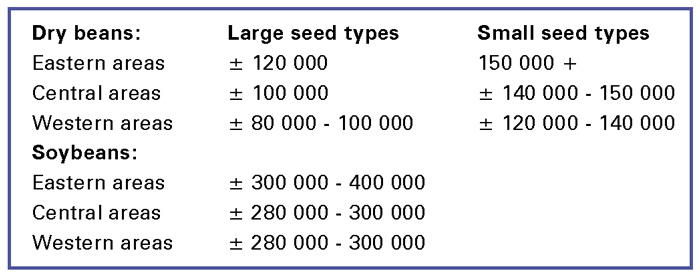5. Now calculate the yield with the following formula:
Plants/10 m ÷ row width x mass/plant (g/plant) ÷ 1 000 = ton/ha
6. Make provision for losses during the harvesting process as follows:
• Adjust the calculated yield for dry beans by a factor of 80%.
• Adjust the calculated yield for soybeans by a factor of 85%.
• Also remember to take the height of the harvester blade into account for soybeans.

Santam Agriculture is constantly working on research to refine the methods for determining accurate hail damage and yield estimates for a range of crops. This research is never regarded as completed. New fields are continuously being studied and existing procedures are tested and adapted to keep pace with, among other things, new cultivars and changed farming techniques.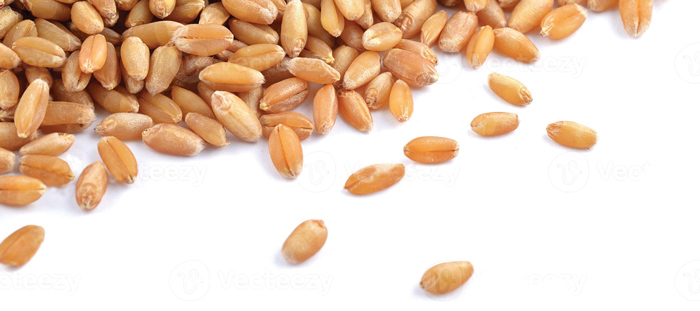Publication: March 2022

Section: Pula/Imvula